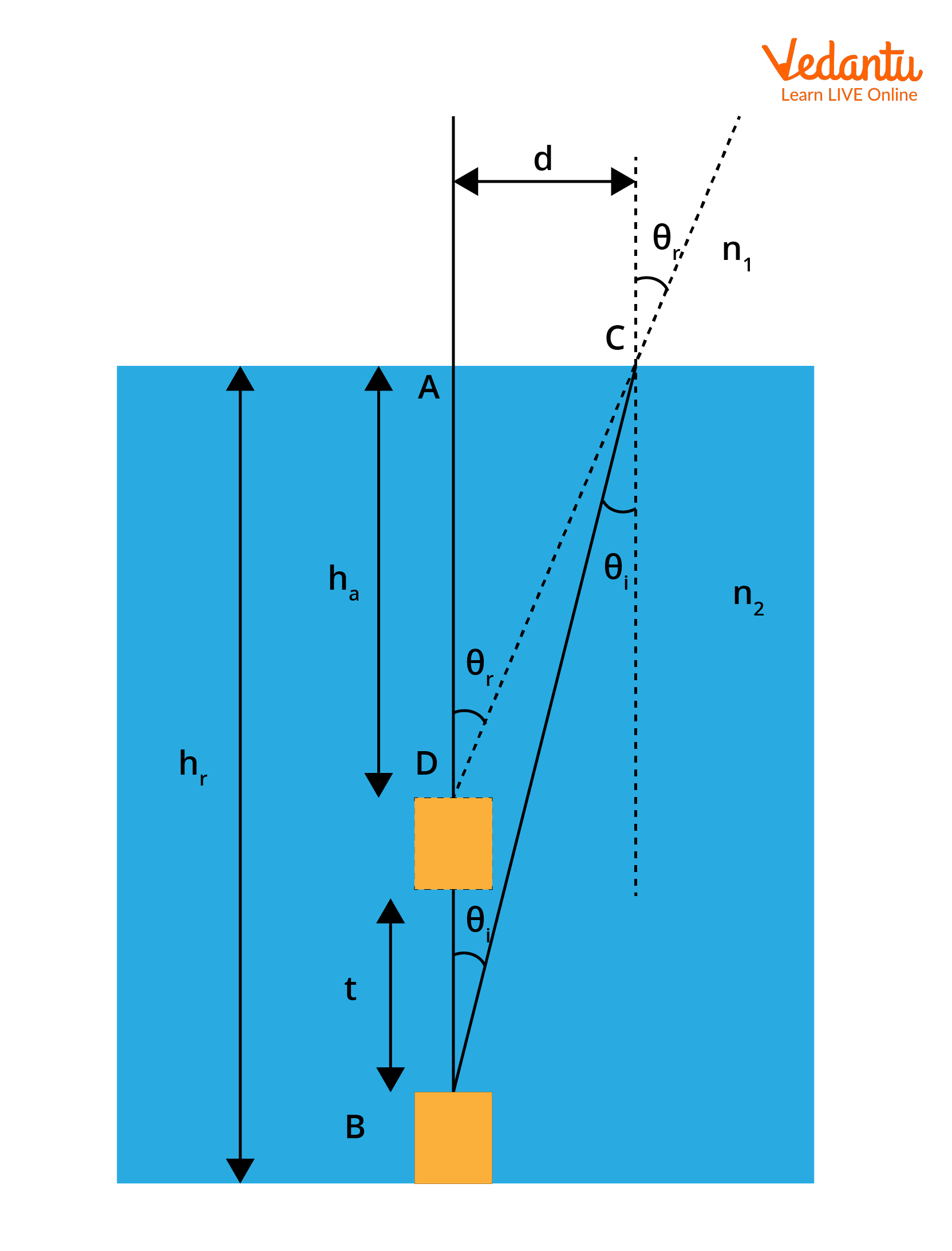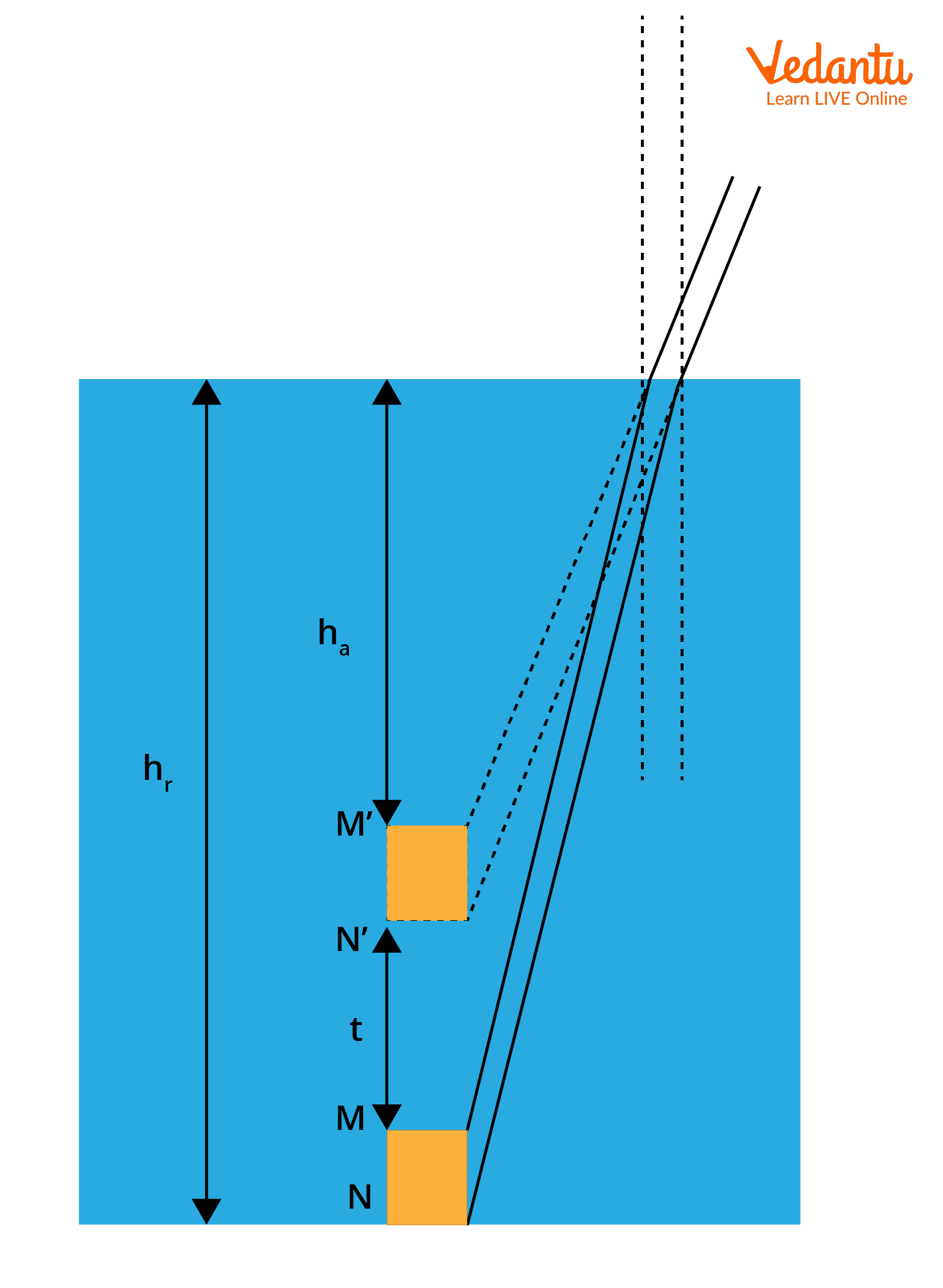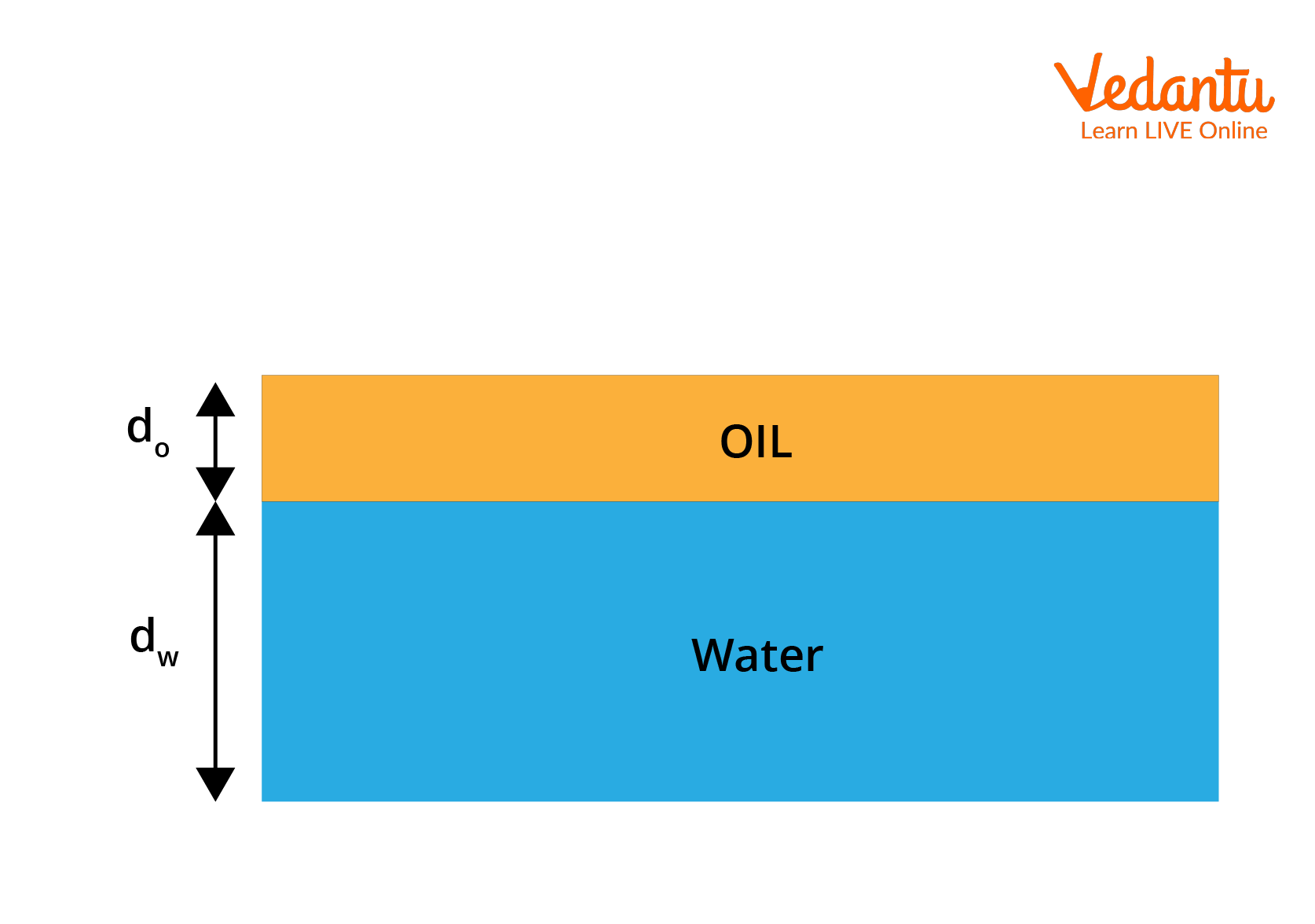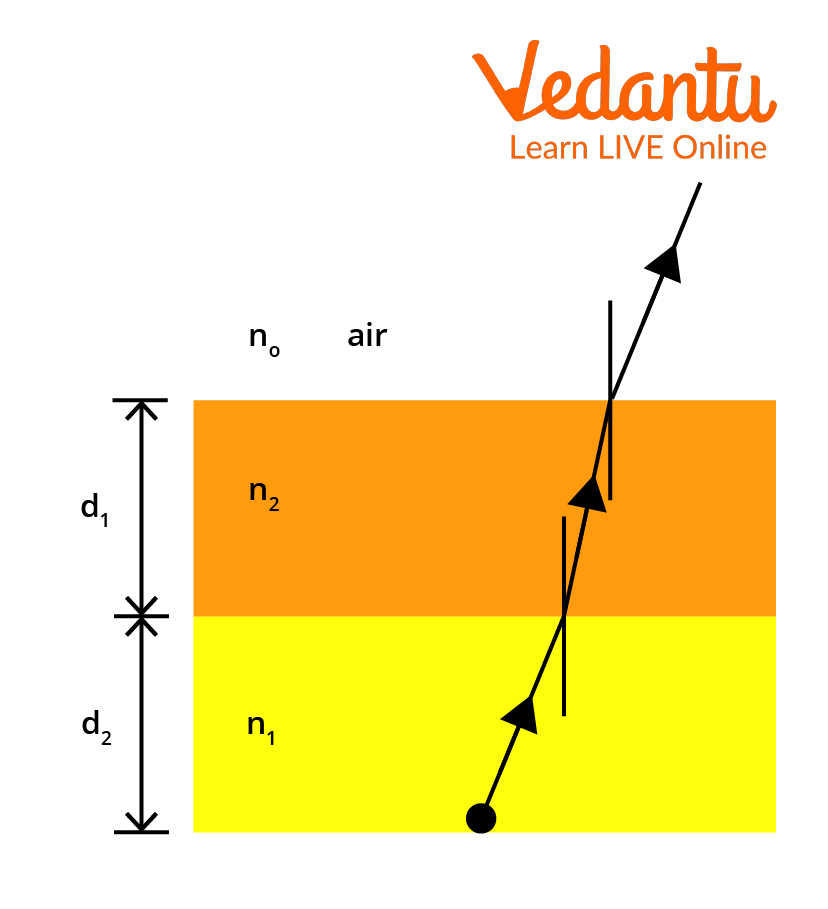Courses
Courses for Kids
Free study material
Offline Centres
More

# Real and Apparent Depth - Important Concept with Solved Examples for JEELast updated date: 08th Dec 2023
Total views: 22.8k
Views today: 0.22k## An Overview of Real Depth and Apparent Depth

Suppose we consider an empty beaker of certain height and we place an object at the base of the beaker. Now, the depth measured is the real depth at which the object is placed. If we put some liquid in the glass, then the measured depth would be the apparent depth. The change in the depth without the change in the position of the object is due to the phenomena of refraction. In this article, we will be understanding this concept.

## What Is Refraction and Refractive Index?

Refraction is a phenomenon in which there is bending of light when it propagates from one medium to the other. From Snell’s law, we know that

1. The incident ray, refracted ray and normal to the interface at the point of incidence lie in the same plane.

2. The ratio of sine of angle of incidence and sine of angle of refraction is constant.

$\dfrac{\sin (i)}{\sin (r)}=n_{21}=\dfrac{n_{2}}{n_{1}}$

Here, i is the angle of incidence and r is the angle of refraction.

n21 is the refractive index of the medium 2 with respect to 1.

When light travels from rarer to denser, the light bends towards the normal. When it travels from denser to rarer, the light bends away from the normal. The amount of bending is governed by Snell’s law. This concept was necessary to understand the concept of apparent depth. Next, we will be discussing the relation between real depth and apparent depth.

## Real Depth and Apparent Depth Derivation and Relation with Refractive Index

Real depth can be defined as the depth at which an object is placed in a beaker or liquid such that the observer and object are in the same medium. The propagation of light from the observer to the object or vice versa should be in the same medium.

Apparent depth can be defined as the depth of the object measured by the observer when the object and observer are in different media. The propagation of light from the observer to the object or vice versa should be in a different medium.

The real depth and apparent depth formula of refractive index can be mathematically derived. Consider a beaker filled with water and a square block placed at the bottom. Now, we measure the position of the square block in the empty glass, which will be the real depth given by the symbol ‘r’ in image 1. We will fill the beaker with a liquid and observe the apparent depth given by ha

The apparent depth will be given as

ha=hr-t.For $\triangle A D C$ we see that

$\tan \left(\theta_{r}\right)=\dfrac{d}{h_{a}}$

For$\triangle A B C$ we see that

$\tan \left(\theta_{i}\right)=\dfrac{d}{h_{r}}$

From Snell’s law, we know

$n_{2} \sin \left(\theta_{i}\right)=n_{1} \sin \left(\theta_{r}\right)$

We will be assuming a paraxial limit; the human eye has a diameter of 4mm - 7mm and the ray can only make small angles with it. So, the Snell’s law equation can be written as

follows:

\begin{align} &n_{2} \tan \left(\theta_{i}\right)=n_{1} \tan \left(\theta_{r}\right) \\ &n_{2} \dfrac{d}{h_{r}}=n_{1} \dfrac{d}{h_{a}} \\ &\dfrac{n_{2}}{n_{1}}=\dfrac{h_{r}}{h_{a}} \end{align}

Here, we see the real depth and apparent depth formula in terms of refractive index. Now, in the next section, we will be discussing other phenomena occurring due to apparent depth.

## Shift in Position and Lateral Magnification

The shift in position of the image with respect to the object can be easily  calculated. It is just the difference between the real depth minus the apparent depth.

$t=h_{r}-h_{a}$

For derivation of lateral magnification:

Consider an object of height ‘t’ placed in the glass beaker as shown in Image 2.

Distance of the image of N from interface is $=\dfrac{n_{2}}{n_{1}} h_{r}$

Distance of the image of N from interface is $=\dfrac{n_{2}}{n}\left(h_{r}-t\right)$

Then height of image observed will be $=\dfrac{n_{2}}{n_{1}} t$## Real Depth and Apparent Depth Numericals

Example 1: A vessel contains water upto the height of 30cm and above it, and oil up to another 20cm of height. The refractive indices of water and oil are 1.33 and 1.30, respectively. Find the apparent depth of the vessel.

Solution:

We know the formula relating real depth, apparent depth and refractive index.

So apparent depth for water and oil  is given by:

\begin{align} &\dfrac{d_{w}}{d_{w^{\prime}}}=\dfrac{1.33}{1.30} \\ &d_{w^{\prime}}=\dfrac{d_{w} \times 1.3}{1.33}=\dfrac{30 \times 1.3}{1.33}=29.32 \end{align}

\begin{align} &\dfrac{d_{o}}{d_{o^{\prime}}}=\dfrac{1.33}{1.30} \\ &d_{o^{\prime}}=\dfrac{d_{o} \times 1.3}{1.33}=\dfrac{20 \times 1.3}{1.33}=19.55 \end{align}

Total apparent depth is given by:

dw+d0=48.87

dw is the real depth in water.

dw’ is the apparent depth in water.

do  is the real depth in oil.

do’ is the apparent depth in oil.Example 2: A bucket of total height 50 cm is half filled with a liquid of refractive index 1.5 and half with another liquid of refractive index 2. Find the apparent depth of the bucket for an observer directly above the bucket.Solution:

Since there are two different media, the apparent depths due to both media have to be added. d1 is the depth of the liquid of refractive index 1.5 and d2 is the depth of liquid with refractive index 2. Since the liquids fill half of the bucket, both d1 and d2 will be 25cm. Now the apparent depth due to both media will be given as

\begin{align} &d=d_{1}\left(\dfrac{n_{0}}{1.5}\right)+d_{2}\left(\dfrac{n_{0}}{2}\right) \\ &d=25\left(\dfrac{1}{1.5}\right)+25\left(\dfrac{1}{2}\right) \\ &d=29.16 \mathrm{~cm} \end{align}

So, the apparent depth is 29.16cm.

## Conclusion

Apparent depth can be said to be a lateral shift in the image of the object due to refraction. As discussed in the article, apparent and real depth are related through refractive index of the medium, where the object is present or what the observer is viewing. The propagation of light from different media leads to refraction and when an observer views the object, they observe a shift in the object from its original position. This phenomena can be seen in nature during sunrise and sunset. Even when the sun has not crossed the horizon, we can see the sun due to the effect of refraction. This can be described as an apparent shift in the position of its image, although the object is not there at position.

## FAQs on Real and Apparent Depth - Important Concept with Solved Examples for JEE

1. What are the factors on which the apparent depth of the object depends?

The apparent depth of the object only depends upon the refractive index of the medium. The refractive index regulates the amount of deviation of light rays coming from the object to the observer’s eye. The refractive index is determined as the ratio of the sine angle of incidence to the sine angle of refraction. The refraction light follows Snell’s law. The refraction of light also matters on behalf of the density of the mediums. The only factor which affects the apparent depth of the object is the refractive index.

2. Why is it important to calculate the critical angle?

In optics, the critical angle is the angle of incidence beyond which the total internal reflection of the light will happen. When the angle of incidence is more than the critical angle, then no refraction will take place. In that case, if a light ray strikes the interface of two different mediums, the incident ray will automatically be reflected into the same medium. It is found to be one of the largest incidence angles for which the reflection of light takes place in the same medium.

3. What is the weightage of this topic in the JEE exam?

The weightage of the topic real and apparent depth is 3%, while the weightage of optics is 6%. This concept is introduced in the section on ray optics which was found as the most important topic for the JEE advanced examination. Nearly 5-6 questions can be expected in the given competitive examination of JEE. It is necessary to practice many questions in preparation for the JEE examination. In this chapter, it is required to keep a check on every topic for better performance in this section.

4. Is real depth always greater than apparent depth? How does the concept of apparent depth help swimmers?

No, real depth is not always greater than apparent depth. In the case of an observer observing the object from a medium whose refractive index is less than the medium in which the object is there, real depth is greater than apparent depth. However, when the observer is viewing from a medium of higher refractive index than the medium in which the object is present, apparent depth is greater than real depth.

Due to the apparent depth, the floor of the swimming pool seems to be less deep than it actually is. Due to which an inexperienced swimmer may drown. However, prior knowledge of it helps in preventing such accidents.

5. If the viewer shifts from normal view then will it affect the correct calculation of apparent depth? What are the factors affecting the apparent depth?

Yes, it does affect the calculation if the observer shifts his/her viewing from normal to a more inclined angle. This is due to the approximations we consider via paraxial limits when deriving the relation between apparent depth, real depth and refractive index. The limit does not allow inclination greater than four degrees. The factors that affect the apparent depth are the refractive index of the medium and the depth upto which the liquid is present.